BlueAdmiral.com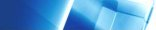Fuzzy Sets

A set is defined by its members. For example:

- the set of all members of class HND2A
- the set of all paid-up members of a snooker club
- the set of all people over 1.8 metres tall
- the set of numbers from 4 to 9 inclusive

Such sets can be represented by Venn diagrams:

 universe of discourse         or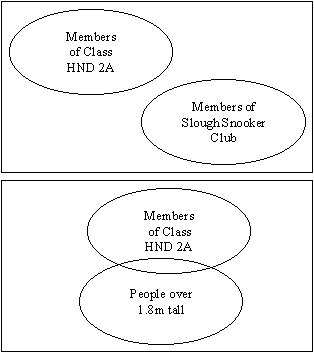Remember that operations may be performed on sets - intersection, union, difference etc.

All the sets mentioned so far have definite boundaries and an object is either a member of the set or it is not. The situation is crisp and clear and such sets are referred to as CRISP sets and they have CRISP boundaries.

Sets may be represented by TRUTH diagrams:
E.g. the set of numbers between 4 and 9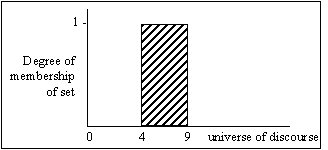The y-axis can be set, arbitrarily, as degree of membership = 0 if the object is not a member of the set and = 1 if it is a member.

This is equivalent to the Boolean algebra TRUE and FALSE.

However, other sets are not so clear. Consider the set of tall people.

Would we call somebody > 2 metres tall?
Would we call somebody > 1.75 metres tall?
Would we call somebody < 1.5 metres tall?

It is clear that the set of 'tall' people does not have a crisp boundary but we all know what we mean by 'tall' and it is possible to discuss such sets while acknowledging that there is a subjective element to the definition of tall. Such a set is called a FUZZY SET and it has FUZZY boundaries - we are unable to say exactly where the dividing line is between 'tall' and 'not tall'.

However, we would normally expect to have some boundaries. There is an upper limit to human height, say 2.2 metres, and a lower limit to the height of adults of, say 1.25 metres. Thus the fuzzy set of 'tall' would be defined over a domain which has boundaries at 1.25 and 2.20 metres. This domain is the range over which we might expect to discuss the height of adults.

The set of tall adults could be represented by the following truth diagram.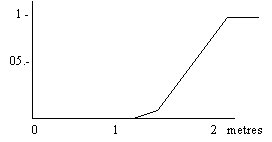Here, objects may have a grade of membership between 0 and 1 with values indicating partial truth. For example we could say that the proposition that somebody who is 1.8 metres high is tall has a truth value of 0.75.

In describing a person's height as 'tall', 'short', 'medium' etc. we are treating height as a linguistic variable which takes the linguistic value of 'tall', 'short' etc. Another example of a linguistic variable would be price used in an expression such as:

The price is low

Again, we are quite happy to use such expressions in everyday speech. In the expression 'The price is low' it is necessary to know what we are talking about - a 'low' price for a diamond ring would not be the same as a low price for a bar of chocolate. Suppose we were discussing the price of a meal in a motorway cafe. We could define the domain for the price of a meal as:

£ sterling 1 2 4 6 8 10 12

On this domain we could then define the fuzzy set 'low' as follows:

 £ 1 2 4 6 8 10 12 Possibilities of Truth Values 1 1 0.7 0.3 0.1 0 0

Note that the figures in the second row are not probabilities (although they may look like probabilities) and they do not sum to one.

This example shows that the definition of the grade of membership of a fuzzy set is not only subjective but also likely to be context dependent - the fuzzy set of 'cheap meal' for example will have a different connotation in McDonalds compared to eating at the Ritz Hotel!

Fuzzy Sets were introduced by Lotfi Zadeh in a paper in 1965 and have important applications in expert systems. For example, in telling someone how to adjust the television sound you would instruct them to turn this knob clockwise a little. You would not say "If the volume <70 decibels then increase the amplification by 8.3%"!!

Fuzzy sets have been used to solve many practical problems such as product pricing or controlling equipment. Mitsubishi have recently introduced a car whose automatic transmission is controlled by fuzzy logic.

Combining fuzzy sets

Just as we can describe the operations intersection, union etc. on crisp sets so it is possible to perform similar operations with fuzzy sets.

Consider the following two fuzzy sets:

(i) the set of small numbers
(ii) the set of numbers near 8

A truth diagram representing these is shown below: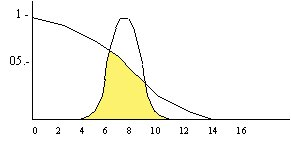The intersection of these two sets is represented by the shaded area and includes those numbers that are both 'small' and 'near
8'.

The greatest truth value (or grade of membership) in the intersection set is less than 1 - there is no number for which it is completely true that it is both 'small' and 'near 8'. How shall we assign truth values for numbers that are in the intersection set? For any particular number in this set i.e. which belongs to both main sets, it seems sensible to assign the smaller of its truth values in the main sets.

Take the number 7, for example. It has a truth value of about
0.3 for its membership of the set of small numbers and a truth
value of about 0.9 for its membership of the set of numbers about
8. Applying the above rule, we would assign it a truth value of
0.3 for its membership of the intersection set of numbers which
are both small and about 8.

Let m represent the degree of membership of a set (truth value)

Then we can write a formula for the intersection of two sets A and B as follows:

m(A AND B) = min {m(A), m(B)}

Truth values can also be regarded as the possibility of an event occurring. The formula makes sense in this case since the possibility of 2 events happening cannot be better than that of the least likely.

Similarly, for the union of two sets, where an object is either in one set or m the other, we will assign the maximum of the corresponding truth values:

m(A OR B) = max {m(A), m(B)}

You may like to check that these formulae also apply to
classical, crisp sets where the truth value for membership of a
set will be either 0 or 1.

One last formula (again, similar to the classic one) concerns the complemention of fuzzy sets:

m(NOT A) = {1 - m(A)}

Use of Fuzzy Sets and Logic in Expert Systems

Some Experts Systems e.g. REVEAL recognize a data type fuzzy set and can manipulate it.

It should be clear from the above notes that fuzziness is an important concept in expert systems. Much knowledge may be expressed in fuzzy terms and it is not always possible to pin the expert down to be more precise.

It is possible to use fuzzy production rules. For example:

If the tomato is red then the tomato is ripe.

Since there are degrees of redness it is not possible to say that every tomato will either be red or not red but only that the clause "The tomato is red" is true to some degree. Hence, the consequent clause can be true to no more than the same degree.

Fuzzy production rules will have an output fuzzy set which must then be translated into an executable action. e.g. what do we do if the tomato has a certain degree of ripeness? Maybe our decision is whether or not to eat it and therefore we need to represent the fuzzy set describing ripeness by a single value. This could then be tested against some threshold value of ripeness so that we could decide whether or not to eat the tomato.

Of course, this is a trivial example. In practice, the output fuzzy set is likely to be more complex but we may still aim at extracting a single value - this process is known as arithmetic defuzzification. An alternative is to translate the fuzzy set into a verbal phrase describing the control action to be taken.